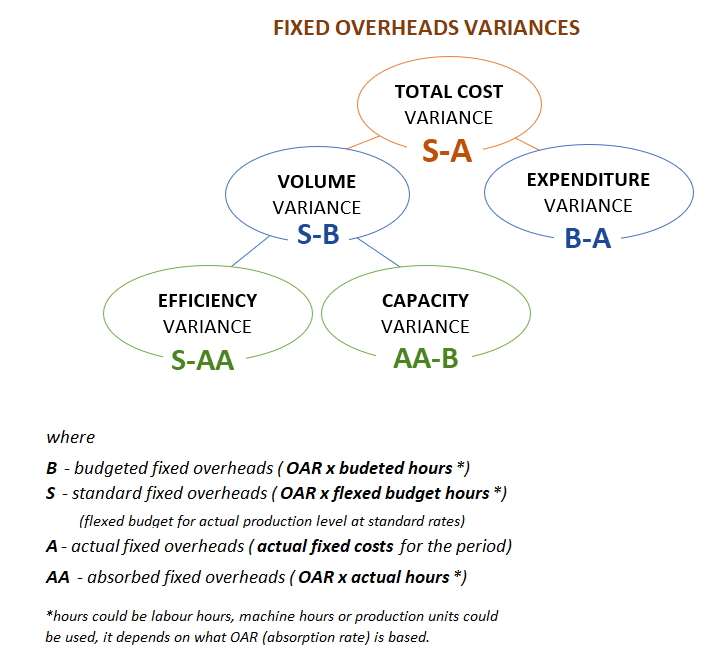Hello,

Wonder if someone could help me with the expenditure variance for the below:

Budgeted production is 10,000 units @ 6 hours per unit with a labour cost of 5 pounds per hour

Actual production is 11,500 units using 70,150 hours at a total cost of 343,735

Overheads are apportioned on the basis of budgeted total labour hours. Total fixed overheads are budgeted at 600,000 with actuals of 630,000

Any general tips on understand these would be appreciated!!

• Hi,

Fixed Overhead Expenditure : Actual - Budget
£630,000 - £600,000 = £30,000

Fixed Overhead Volume : Budget - SH * SR (Standard Hour * Standard Rate)
£600,000 - 300,000 (10,000*6*5) = 300,000

Fixed Overhead Capacity : Budget - AH * SR (Actual Hour * Standard Rate)
£600,000 - 350750 (70150*5) = 249250

Fixed Overhead Efficiency: AH * SR - SH * SR (Standard Hour * Standard Rate)
350750 - 300,000 = 50,750

Total Fixed Overhead : Actual - SH * SR (Standard Hour * Standard Rate)
£630,000 - 300,000 = 330,000
•• OAR = 600,000/60,000 = £10/labour hour
B= £600,000
S=£10 x (11500 x 6 h) = £690,000
A=£630,000
AA=£10 x 70,150= £701,500